## Example Questions

← Previous 1 3 4 5

### Example Question #1 : How To Solve For A Variable As Part Of A Fraction

Solve for.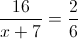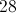Explanation:

Cross multiply.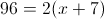Dsitribute.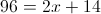Solve for.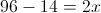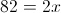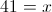### Example Question #2 : How To Solve For A Variable As Part Of A Fraction

The quotient of a fraction is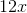. If the numerator is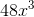, what is the value of the denominator?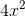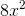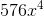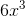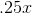Explanation:

Step 1: Set up the equation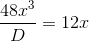Step 2: Solve for D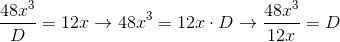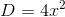### Example Question #3 : How To Solve For A Variable As Part Of A Fraction

Solve for: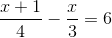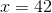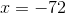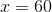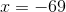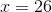Explanation:

Solve for x:Step 1: Find the least common denominator,, and adjust the fractions accordingly: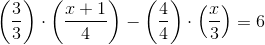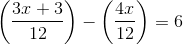Solve for: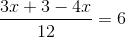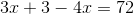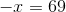### Example Question #1 : How To Solve For A Variable As Part Of A Fraction

If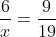, then what is the value of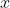?

7/12

none of these

9/114

3/38

38/3

38/3

Explanation:

cross multiply:

(6)(19) = 9x

114=9x

x = 38/3

### Example Question #1571 : Gre Quantitative Reasoning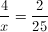Find x.

None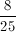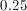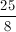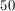Explanation:

Cross multiply: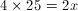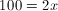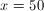### Example Question #2 : How To Solve For A Variable As Part Of A Fraction

The numerator of a fraction is the sum of 4 and 5 times the denominator. If you divide the fraction by 2, the numerator is 3 times the denominator. Find the simplified version of the fraction.Explanation:

Let numerator = N and denominator = D.

According to the first statement,

N = (D x 5) + 4.

According to the second statement, N / 2 = 3 * D.

Let's multiply the second equation by –2 and add itthe first equation:

–N = –6D

+[N = (D x 5) + 4]

=

–6D + (D x 5) + 4 = 0

–1D + 4 = 0

D = 4

Thus, N = 24.

Therefore, N/D = 24/4 = 6.

### Example Question #7 : How To Solve For A Variable As Part Of A Fraction

Solve the following equation for the given variable: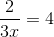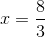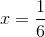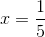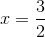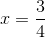Explanation:

To solve this equation we have to multiply both sides by the denominator to get rid of the fraction.

Doing this yields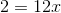Then to solve the last step is to isolate the variable by dividing both sides by 12.
Thus,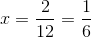.

### Example Question #8 : How To Solve For A Variable As Part Of A Fraction

For what value ofis the equation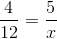true?Explanation:

When the equation is cross multiplied, it becomes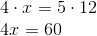.

Hence,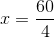, or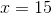### Example Question #9 : How To Solve For A Variable As Part Of A Fraction

Solve the following equation for: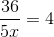.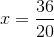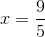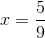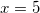Explanation:

To solve an equation with a variable in a fraciton, treat the denominator as a constant value and multiply both sides of the equation by the denominator in order to eliminate it.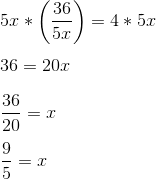### Example Question #10 : How To Solve For A Variable As Part Of A Fraction

Solve for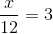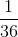To find the answer, multiply the right side by. The result is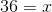.